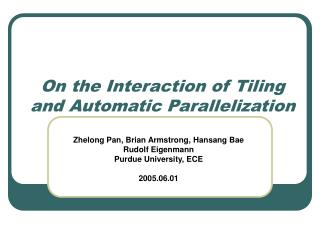# On the Interaction of Tiling and Automatic Parallelization - PowerPoint PPT PresentationDownload PresentationOn the Interaction of Tiling and Automatic Parallelization

On the Interaction of Tiling and Automatic Parallelization
Download Presentation## On the Interaction of Tiling and Automatic Parallelization

- - - - - - - - - - - - - - - - - - - - - - - - - - - E N D - - - - - - - - - - - - - - - - - - - - - - - - - - -
##### Presentation Transcript

1. On the Interaction of Tiling and Automatic Parallelization Zhelong Pan, Brian Armstrong, Hansang Bae Rudolf Eigenmann Purdue University, ECE 2005.06.01

2. Outline • Motivation • Tiling and Parallelism • Tiling in concert with parallelization • Experimental results • Conclusion

3. Parallelizing Compiler Backend Compiler Tiled OpenMP Program Executable Source Code Motivation • Apply tiling in a parallelizing compiler (Polaris) • Polaris generates parallelized programs in OpenMP • Backend compilers generate executable • Investigate performance on real benchmarks

4. Issues • Tiling interacts with parallelization passes • Data dependence test, induction, reduction, … • Load balancing is necessary • Parallelism and locality are compromised

5. Outline • Motivation • Tiling and parallelism • Tiling in concert with parallelization • Experimental results • Conclusion

6. Tiling • Loop strip-mining • Li strip-mined into Li’ and Li’’ • Cross-strip loops: Li’ • In-strip loops: Li’’ • Loop permutation (a) Matrix Multiply DO I = 1, N DO K = 1, N DO J = 1, N Z(J,I) = Z(J,I) + X(K,I) * Y(J,K) (b) Tiled Matrix Multiply DO K2 = 1, N, B DO J2 = 1, N, B DO I = 1, N DO K1 = K2, MIN(K2+B-1,N) DO J1 = J2, MIN(J2+B-1,N) Z(J1,I) = Z(J1,I) + X(K1,I) * Y(J1,K1)

7. Possible Approaches • Tiling before parallelization • Possible performance degradation • Tiling after parallelization • Possible wrong result • Our approach • Tiling in concert with parallelization

8. 3 4 2 1 In-strip loop 2 4 1 3 cross-strip dependence, “<=” Cross-strip loop cross-strip dependence, “<<” Direction Vector after Strip-mining Lemma. Strip-mining may create more direction vectors, i.e. =  ==, <  =< or <*, >  => or >* “<“ in-strip dependence, “=<” cross-strip dependence, “<>”

9. L2 DO L1 = 1, 4 DO L2 = 1, 4 A(L1,L2) = A(L1+1,L2+1) S P S DO LC1 = 1, 4, 2 DO LC2 = 1, 4, 2 DO LI1 = LC1, LC1+1, 1 DO LI2 = LC2, LC2+1, 1 A(LI1,LI2) = A(LI1+1,LI2+1) S A Tile S L1 P Parallelism after Tiling Theorem. After tiling, the in-strip loops have the same parallelism as the original ones, but some cross-strip loops may change to serial. “<” makes the corresponding cross-strip loop serial. Tiling after parallelization is unsafe

10. Outline • Motivation • Tiling and Parallelism • Tiling in concert with parallelization • Experimental results • Conclusion

11. Trading off Parallelism and Locality • Enhancing locality may reduce parallelism • Tiling may change fork-join overhead • [SP]  [SSP], increase fork-join overhead. • [SP]  [PSP], decrease fork-join overhead. • [PS]  [SPS], increase fork-join overhead. • [SS]  [SSS], no change of fork-join overhead. • [PP]  [PPP], no change of fork-join overhead. DO J=1,N DO I=1,N A(I,J) = A(I,J+1)

12. . . . (c) In-strip loop is parallel. Machine has distributed caches. RefT = CS * P … (d) Cross-strip loop is parallel. Machine has a shared cache. RefT = CS / P (b) Cross-strip loop is parallel. Machine has distributed caches. RefT = CS (a) Loop is sequential. RefT = CS (e) In-strip loop is parallel. Machine has a shared cache. RefT = CS Tile Size Selection • Data references in a tile should be close to the cache size. Cache Tile RefT : Mem ref. in a tile CS: Cache size P: # of Proc.

13. T = 80 P = 4 I = 512  S = 64 Load Balancing • Balance the parallel cross-strip loop (a) Before tiling (balanced) DO I = 1, 512 DO J = 1, 512 (b) After tiling (not balanced) DO J1 = 1, 512, 80 DO I = 1, 512 DO J = 1, MIN(J1+79,512) • Balanced tile size S: Balanced tile size T: Tile size by LRW P: Number of processors I: Number of iterations

14. Impact on parallelization passes • Tiling does not change the loop body • Limited effect on parallelization passes • Induction variable substitution • Privatization • Reduction variable recognition

15. Tiling in Concert with Parallelization • Find the best tiled version in favor of • parallelism first and then locality • Compute tile size based on • parallelism and cache configuration • Tune the tile size to balance load • Update reduction/private variable attribute • Generate two versions if iteration number I unknown: • Original parallel version is used when I is small • Otherwise, tiled version is used

16. Outline • Motivation • Tiling and Parallelism • Tiling in concert with parallelization • Experimental results • Conclusion

17. Result on SPEC CPU 95

18. Result on SPEC CPU 2000

19. On the performance bound Percentage of tilable loops based on reuse

20. Conclusion • Tiling and parallelism • Tiling in concert with parallelization • Comprehensive evaluation